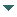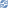# gvSIG

##### Personal tools
You are here: Home Curve
gvSIG DesktopgvSIG Desktop

Cached time 11/21/13 11:24:39# Curve

Up one level
 ◄ Previous Point Next: Arc ►

To create a curve we can use the generic method create from the manager. To establish the coordenate's values we can use an object of the GeneralPathX type that is also available because the backward compatibility. The steps to create a polyline from the point 5,5 to the 10,10 are this:

```Curve curve = (Curve)geometryManager.create(TYPES.CURVE, SUBTYPES.GEOM2D);
GeneralPathX generalPathX = new GeneralPathX();
generalPathX.moveTo(5, 5);
generalPathX.lineTo(10, 10);
curve.setGeneralPath(generalPathX);
```

A curve must be created from the own model's objects. In the curve case, they will be Point type objects. The following code let us to create a curve with the same coordentes like the previous example:

```Point point1 = geometryManager.createPoint(5, 5, SUBTYPES.GEOM2D);
Point point2 = geometryManager.createPoint(10, 10, SUBTYPES.GEOM2D);
Curve curve = (Curve)geometryManager.create(TYPES.CURVE, SUBTYPES.GEOM2D);
```

The addVertex method inserts a new vertex in the last position in a Curve object and it should be used in the geometry creation. In the other hand, there're more methods which let us the edition of the curve's coordenates. For example, if we want to change the final point of the curve in the last example, we could do this:

```Point endPoint = geometryManager.createPoint(15, 15, SUBTYPES.GEOM2D);
curve.setVertex(1, endPoint);
```

The setVertex method fixes the value of one of the internal points which are part of the curve composition and replace it for the point which was previously. In the other hand, we can also insert a point inside the curve. For example, to insert the point 7,7 in the second position we must execute the following code:

```Point middlePoint = geometryManager.createPoint(7, 7, SUBTYPES.GEOM2D);
curve.insertVertex(1, middlePoint);
```

The first parameter is the point position that we want to put in. In this case, the resultant curve will have the coordinates 5,5 7,7 and 15,15 such as we have been defining.

 ◄ Previous Point Next: Arc ►